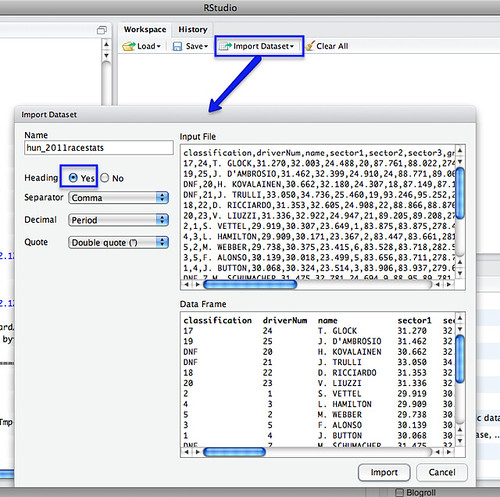# Merging Two Different Datasets Containing a Common Column With R and R-Studio

Another way for the database challenged (such as myself!) for merging two datasets that share at least one common column…

This recipe using the cross-platform stats analysis package, R. I use R via the R-Studio client, which provides an IDE wrapper around the R environment.

So for example, here’s how to merge a couple of files sharing elements in a common column…

First, load in your two data files – for example, I’m going to load in separate files that contain qualifying and race stats from the last Grand Prix:We can merge the datasets using a command of the form:

m=merge(hun_2011racestats,hun_2011qualistats,by="driverNum")

The by parameter identifies which column we want to merge the tables around. (If the two datasets have different column names, you need to set by.x= and by.y= to specify the column from each dataset that is the focus for merging).

So for example, in the simple case where we are merging around two columns of the same name in different tables:

After the merge, column names for columns from the first table have the .x suffix added, and from the second, .y.

We can then export the combined dataset as a CSV file:

write.csv(m, file = "demoMerge.csv")[If you want to extract a subset of the columns, specify the required columns in an R command of the form: m2=m[c(“driverNum”,”name.x”,”ultimate.x”,”ultimate.y”)] See also: R: subset]

Simples;-)

PS in the above example, the merge table only contains merged rows. If there are elements in the common column of one table, but not the other, that partial data will not be included in the merged table. To include all rows, set all=TRUE. To include all rows from the first table, but not unmatched rows from the second, set all.x=TRUE; (the cells from columns in the unmatched row of the second table will be set to NA). (all.y=TRUE is also legitimate). From the R merge documentation:

In SQL database terminology, the default value of all = FALSE [the default] gives a natural join, a special case of an inner join. Specifying all.x = TRUE gives a left (outer) join, all.y = TRUE a right (outer) join, and both (all=TRUE a (full) outer join. DBMSes do not match NULL records, equivalent to incomparables = NA in R.

For other ways of combining data from two different data sets, see:
Merging Datasets with Common Columns in Google Refine
A Further Look at the Orange Data Playground – Filters and File Merging
Merging CSV data files with Google Fusion Tables

If you know of any other simple ways of joining data files about a common column, please reveal all in the comments:-)